The mathematical expression for magnetic flux density was derived by Jean Baptiste Biot and Felix Savart. Talking the deflection of a c...

The mathematical expression for magnetic flux density was derived by Jean Baptiste Biot and Felix Savart. Talking the deflection of a compass needle as a measure of the intensity of a current, varying in magnitude and shape, the two scientists concluded that any current element projects into space a magnetic field, the magnetic flux density of which dB, is directly proportional to the length of the element dl, the current I, the sine of the angle and θ between direction of the current and the vector joining a given point of the field and the current element and is inversely proportional to the square of the distance of the given point from the current element, r. This is Biot Savart law statement.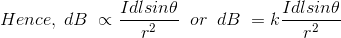Where, K is a constant, depends upon the magnetic properties of the medium and system of the units employed. In SI system of unit,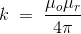Therefore, final Biot Savart law derivation is,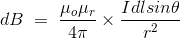Let us consider a long wire carrying an current I and also consider a point p. The wire is presented in the below picture by red color. Let us also consider an infinitely small length of the wire dl at a distance r from the point P as shown. Here, r is a distance vector which makes an angle θ with the direction of current in the infinitesimal portion of the wire.
As the current through that infinitesimal length of wire is same as the current carried by the wire itself.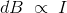It is also very natural to think that the magnetic field density at that point P due to that infinitesimal length dl of wire is inversely proportional to the square of the straight distance from point P to center of dl. That means distance r of this infinitesimal portion of the wire is increased the magnetic field density due to this infinitesimal length of wire, at point P decreases and if the distance of this portion of wire from point P, is decreased, the magnetic field density at point P due to this infinitesimal length of wire increases accordingly.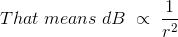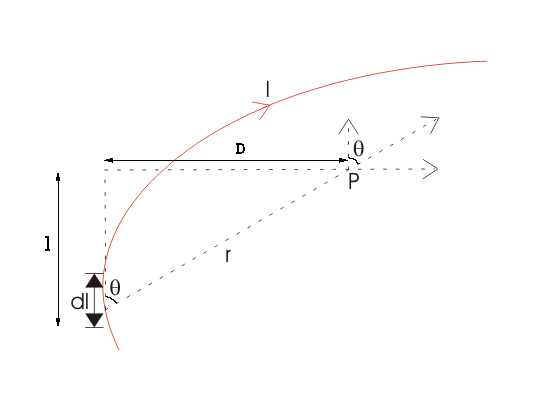Lastly, field density at that point P due to that infinitesimal portion of wire is also directly proportional to the actual length of the infinitesimal length dl of wire. As θ be the angle between distance vector r and direction of current through this infinitesimal portion of the wire. The component of dl directly facing perpendicular to the point P is dlsinθ,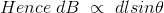Now combining these three statements, we can write,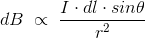This is the basic form of Biot Savart's Law
Now putting the value of constant k (which we have already introduced at the beginning of this article) in the above expression, we get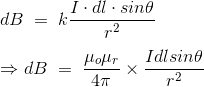Here, μ0 used in the expression of constant k is absolute permeability of air or vacuum and it's value is 4π10-7 Wb/ A-m in SI system of units. μr of the expression of constant k is relative permeability of the medium.
Now, flux density(B) at the point P due to total length of the current carrying conductor or wire can be represented as,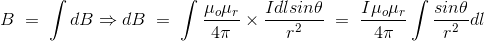If D is the perpendicular distance of the point P form the wire, then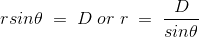Now, the expression of flux density B at point P can be rewritten as,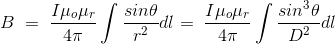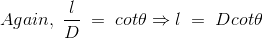As per the figure above,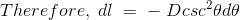Finally the expression of B comes as,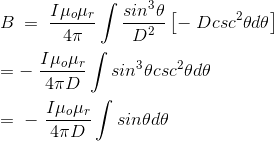This angle θ depends upon the length of the wire and the position of the point P. Say for certain limited length of the wire, angle θ as indicated in the figure above varies from θ1 to θ2. Hence, flux density at point P due to total length of the conductor is,Let's imagine the wire is infinitely long, then θ will vary from 0 to π that is θ1 = 0 to θ2 = π. Putting these two values in the above final expression of Biot Savart law, we get,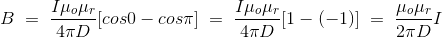This is nothing but the expression of Ampere's Law.

If you try to visualize the condition, you can easily understand the magnetic field density at that point P due to that infinitesimal length dl of wire is directly proportional to current carried by this portion of the wire. That means current through this infinitesimal portion of the wire is increased the magnetic field density due to this infinitesimal length of wire, at point P increases proportionally and if the current through this portion of wire is decreased the magnetic field density at point P due to this infinitesimal length of wire decreases proportionally.Name

BASIC ELECTRICAL,12,BATTERIES,4,CIRCUIT THEORIES,9,CONTROL SYSTEMS,3,DC MOTOR,1,DIGITAL ELECTRONICS,1,DISTRIBUTED GENERATION,2,DISTRIBUTION,6,ELECTRICAL DRIVES,1,ELECTRICAL LAWS,8,ELECTRONICS DEVICES,2,General,7,GENERATION,3,GENERATOR,1,HIGH VOLTAGE,4,ILLUMINATION,1,INDUCTION MOTOR,7,MATERIALS,1,MEASUREMENT,1,MOTOR,1,POWER ELECTRONICS,2,PROJECTS ON INDUCTION MOTOR,1,PROTECTION,1,SMART GRID,3,SWITCHGEAR,4,SYNCHRONOUS MOTOR,1,TRANSFORMER,6,TRANSMISSION,4,
ltr
item
Electrical for Us: Biot Savart Law
Biot Savart Law
http://electrical4u.com/electrical/basic-electrical-equation/biot-savart-law-1.gif
Electrical for Us
https://www.sanjaysah.com.np/2016/11/biot-savart-law.html
https://www.sanjaysah.com.np/
http://www.sanjaysah.com.np/
http://www.sanjaysah.com.np/2016/11/biot-savart-law.html
true
3851448774078769448
UTF-8